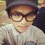# The Mystery Behind the Quadratic Formula

In Quadratic, there are lots of formula you should remember (or else it would be difficult for you) . I'm sure that you guys have already known this formula :

$$x=\frac { -b\pm \sqrt { { b }^{ 2 }-4ac } }{ 2a }$$

The question is, do you know that from the general form $ax^{ 2 }{ +bx }+c=0$ you can form the quadratic formula as shown above ? It seems not important for the beginners, but it really helps to improve our understanding in mathematics and we consider it as our general knowledge . Let me show you how it works :)

$Step\quad 1:$ Rearrange the general form in the term of $a{ x }^{ 2 }+{ bx }=-c$

$Step\quad 2:$ Since $a>0$, we can divide both side by $a$ to get ${ x }^{ 2 }+\frac { b }{ a } x=-\frac { c }{ a }$

$Step\quad 3:$ Since the coefficient of ${ x }^{ 2 }$ is 1, we can use the "Completing The Square" method to complete the square on the left side by adding the square of $\frac { 1 }{ 2 }$ of the coefficient of $x$ ; $(\frac { 1 }{ 2 } \times \frac { b }{ a } )^{ 2 }=\frac { { b }^{ 2 } }{ { 4a }^{ 2 } }$

$Step\quad 4:$ Add the product in $Step\quad 3$ to both side, then ${ x }^{ 2 }+\frac { b }{ a } x+\frac { { b }^{ 2 } }{ { 4a }^{ 2 } } =\frac { { b }^{ 2 } }{ { 4a }^{ 2 } } -\frac { c }{ a }$

$Step\quad 5:$ Factorise the left side completely to get $(x+\frac { b }{ 2a } )^{ 2 }$

$Step\quad 6:$ Solve the Right-side of the equation:

$=\frac { { b }^{ 2 } }{ { 4a }^{ 2 } } -\frac { c }{ a } \\ =\frac { { b }^{ 2 } }{ { 4a }^{ 2 } } -\frac { 4ac }{ { 4a }^{ 2 } } \\ =\frac { { b }^{ 2 }-4ac }{ { 4a }^{ 2 } }$

$Step\quad 7:$ Combine the Left-side and Right-side of the equation to get

$(x+\frac { b }{ 2a } )^{ 2 }=\quad \frac { { b }^{ 2 }-4ac }{ { 4a }^{ 2 } } \\ x+\frac { b }{ 2a } =\quad \pm \sqrt { \frac { { b }^{ 2 }-4ac }{ { 4a }^{ 2 } } } \\ x+\frac { b }{ 2a } =\quad \frac { \pm \sqrt { { b }^{ 2 }-4ac } }{ 2a } \\ x\quad =\quad -\frac { b }{ 2a } \pm \frac { \sqrt { { b }^{ 2 }-4ac } }{ 2a } \\ x\quad =\quad \frac { -b\pm \sqrt { { b }^{ 2 }-4ac } }{ 2a } \$/extract_itex] You don't have to memorize all this 7 steps. All you need is to understand every solution in each step. Use your algebraic skills to solve the general form. Practice makes perfect. Trust me, it will improve your algebraic skills.Note by Haqkiem Learner 6 years, 7 months ago This discussion board is a place to discuss our Daily Challenges and the math and science related to those challenges. Explanations are more than just a solution — they should explain the steps and thinking strategies that you used to obtain the solution. Comments should further the discussion of math and science. When posting on Brilliant: • Use the emojis to react to an explanation, whether you're congratulating a job well done , or just really confused . • Ask specific questions about the challenge or the steps in somebody's explanation. Well-posed questions can add a lot to the discussion, but posting "I don't understand!" doesn't help anyone. • Try to contribute something new to the discussion, whether it is an extension, generalization or other idea related to the challenge. • Stay on topic — we're all here to learn more about math and science, not to hear about your favorite get-rich-quick scheme or current world events. MarkdownAppears as *italics* or _italics_ italics **bold** or __bold__ bold - bulleted- list • bulleted • list 1. numbered2. list 1. numbered 2. list Note: you must add a full line of space before and after lists for them to show up correctly paragraph 1paragraph 2 paragraph 1 paragraph 2 [example link](https://brilliant.org)example link > This is a quote This is a quote  # I indented these lines # 4 spaces, and now they show # up as a code block. print "hello world" # I indented these lines # 4 spaces, and now they show # up as a code block. print "hello world" MathAppears as Remember to wrap math in $$ ... $$ or \[ ... $ to ensure proper formatting.
2 \times 3 $2 \times 3$
2^{34} $2^{34}$
a_{i-1} $a_{i-1}$
\frac{2}{3} $\frac{2}{3}$
\sqrt{2} $\sqrt{2}$
\sum_{i=1}^3 $\sum_{i=1}^3$
\sin \theta $\sin \theta$
\boxed{123} $\boxed{123}$